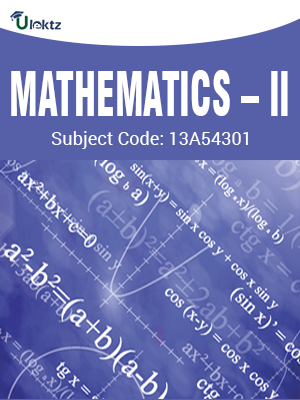•My WalletMy Order
•My Profile
•My Connections
•My Books
•My Videos
•My Tests
•My Calender
•My Messages
•My Shopping Cart
•My Orders
•Account Settings
•Help

# Book Details# MATHEMATICS – II

 Course Code : 13A54301 Author : uLektz University : Jawaharlal Nehru Technological University, Anantapur (JNTUA) Regulation : 2013 Categories : Civil Format :ePUB3 (DRM Protected) Type : eBook

FREE

Description :MATHEMATICS – II of 13A54301 covers the latest syllabus prescribed by Jawaharlal Nehru Technological University, Anantapur (JNTUA) for regulation 2013. Author: uLektz, Published by uLektz Learning Solutions Private Limited.

Note : No printed book. Only ebook. Access eBook using uLektz apps for Android, iOS and Windows Desktop PC.

##### Topics
###### UNIT I:COMPLEX MATRICES

1.1 Rank – Echelon form, normal form – Consistency of System of Linear equations

1.2 Linear transformations Hermitian - Skew-Hermitian and Unitary matrices and their properties

1.3 Eigen Values, Eigen vectors for both real and complex matrices

1.4 Cayley – Hamilton Theorem and its applications - Diagonolization of matrix - Calculation of powers of matrix

1.5 Quadratic forms - Reduction of quadratic form to canonical form and their nature

###### UNIT II:Solution of Algebraic and Transcendental Equations

2.1 Introduction - The Method of False Position - Newton-Raphson Method

2.2 Interpolation:-Introduction - Newton’s forward and backward interpolation formulae - Lagrange’s Interpolation formula

2.3 Curve fitting: Fitting a straight line - Second degree curve - Exponentional curve-Power curve by method of least squares

###### UNIT III:Numerical Differentiation and Integration

3.1 Numerical Differentiation and Integration - Trapezoidal rule - Simpson’s 1/3 Rule – Simpson’s 3/8 Rule

3.2 Numerical solution of Ordinary Differential equations: Solution by Taylor’s series - Picard’s Method of successive Approximations

3.3 Euler’s Method - Runge-Kutta Method - Predictor-Corrector Method - Milne’s Method

###### UNIT IV:Fourier Series

4.1 Determination of Fourier coefficients - Fourier series - Even and odd functions - Fourier series in an arbitrary interval

4.2 Even and odd period, continuation - Half-range Fourier sine and cosine expansions

4.3 Fourier integral theorem - sine and cosine integrals

4.4 Fourier transforms - Fourier sine and cosine transforms – Properties - Inverse transforms – Finite Fourier transforms

###### UNIT V:Partial differential equations

5.1 Formation of partial differential equations by elimination of arbitrary constants and arbitrary functions

5.2 Method of separation of variables - Solutions of one dimensional wave equation, heat equation and two-dimensional Laplace’s equation under initial and boundary conditions

### Related Books